# Hermite-Hadamard inequality for functions whose derivatives absolute values are preinvex

## Abstract

In this article, we extend some estimates of the right-hand side of a Hermite-Hadamard-type inequality for preinvex functions. Then, a generalization to functions of several variables on invex subsets of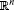is introduced.

## 1 Introduction and preliminary

Let $I=\left[c,d\right]$ be an interval on the real line , let $f:I\to \mathbb{R}$ be a convex function and let $a,b\in \left[c,d\right]$, $a. We consider the well-known Hadamard’s inequality

$f\left(\frac{a+b}{2}\right)\le \frac{1}{b-a}{\int }_{a}^{b}f\left(x\right)\phantom{\rule{0.2em}{0ex}}dx\le \frac{f\left(a\right)+f\left(b\right)}{2}.$
(1)

Both inequalities hold in the reversed direction if f is concave. We note that Hadamard’s inequality may be regarded as a refinement of the concept of convexity and it follows easily from Jensen’s inequality. Hadamard’s inequality for convex functions has received renewed attention in recent years and a remarkable variety of refinements and generalizations have been found (see, for example, ).

The classical Hermite-Hadamard inequality provides estimates of the mean value of a continuous convex function $f:\left[a,b\right]\to \mathbb{R}$.

Dragomir and Agarwal  used the formula,

$\frac{f\left(a\right)+f\left(b\right)}{2}-\frac{1}{b-a}{\int }_{a}^{b}f\left(x\right)\phantom{\rule{0.2em}{0ex}}dx=\frac{b-a}{2}{\int }_{0}^{1}\left(1-2t\right){f}^{\mathrm{\prime }}\left(ta+\left(1-t\right)b\right)\phantom{\rule{0.2em}{0ex}}dt$
(2)

to prove the following results.

Theorem 1.1 Assume $a,b\in \mathbb{R}$ with $a and $f:\left[a,b\right]\to \mathbb{R}$ is a differentiable function on $\left(a,b\right)$. If $|{f}^{\prime }|$ is convex on $\left[a,b\right]$ then the following inequality holds true

$|\frac{f\left(a\right)+f\left(b\right)}{2}-\frac{1}{b-a}{\int }_{a}^{b}f\left(x\right)\phantom{\rule{0.2em}{0ex}}dx|\le \frac{\left(b-a\right)\left(|{f}^{\prime }\left(a\right)|+|{f}^{\prime }\left(b\right)|\right)}{8}.$
(3)

Theorem 1.2 Assume $a,b\in \mathbb{R}$ with $a and $f:\left[a,b\right]\to \mathbb{R}$ is a differentiable function on $\left(a,b\right)$. Assume $p\in \mathbb{R}$ with $p>1$. If ${|{f}^{\prime }|}^{\frac{p}{p-1}}$ is convex on $\left[a,b\right]$ then the following inequality holds true

$|\frac{f\left(a\right)+f\left(b\right)}{2}-\frac{1}{b-a}{\int }_{a}^{b}f\left(x\right)\phantom{\rule{0.2em}{0ex}}dx|\le \frac{b-a}{2{\left(p+1\right)}^{1/p}}\cdot {\left[\frac{{|{f}^{\prime }\left(a\right)|}^{\frac{p}{p-1}}+{|{f}^{\prime }\left(b\right)|}^{\frac{p}{p-1}}}{2}\right]}^{\frac{p-1}{p}}.$
(4)

Ion  presented some estimates of the right-hand side of a Hermite-Hadamard-type inequality in which some quasi-convex functions are involved.

In recent years, several extensions and generalizations have been considered for classical convexity. A significant generalization of convex functions is that of invex functions introduced by Hanson . Weir and Mond  introduced the concept of preinvex functions and applied it to the establishment of the sufficient optimality conditions and duality in nonlinear programming. Aslam Noor [9, 10] introduced the Hermite-Hadamard inequality for preinvex and log-preinvex functions.

In this article, we generalize the results in  for functions whose first derivatives absolute values are preinvex. Also some results for functions whose second derivatives absolute values are preinvex will be given. Now, we recall some notions in invexity analysis which will be used throughout the article (see [11, 12] and references therein).

Definition 1.1 A set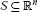is said to be invex with respect to the map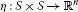, if for every $x,y\in S$ and $t\in \left[0,1\right]$,

$y+t\eta \left(x,y\right)\in S.$
(5)

It is obvious that every convex set is invex with respect to the map $\eta \left(x,y\right)=x-y$, but there exist invex sets which are not convex (see ). Letbe an invex set with respect to. For every $x,y\in S$ the η-path ${P}_{xv}$ joining the points x and $v:=x+\eta \left(y,x\right)$ is defined as follows

${P}_{xv}:=\left\{z:z=x+t\eta \left(y,x\right):t\in \left[0,1\right]\right\}.$

Definition 1.2 Letbe an invex set with respect to. Then, the functionis said to be preinvex with respect to η, if for every $x,y\in S$ and $t\in \left[0,1\right]$,

$f\left(y+t\eta \left(x,y\right)\right)\le tf\left(x\right)+\left(1-t\right)f\left(y\right).$
(6)

Every convex function is a preinvex with respect to the map $\eta \left(x,y\right)=x-y$ but the converse does not holds. For properties and applications of preinvex functions, see [12, 13] and references therein.

The organization of the article is as follows: In Section 2, some generalizations of Hermite-Hadamard-type inequality for first-order differentiable functions are given. Section 3 is devoted to a generalization to several variable preinvex functions. Hermite-Hadamard-type inequality for second-order differentiable functions are studied in Section 23.

## 2 First-order differentiable functions

In this section, we introduce some generalizations of Hermite-Hadamard-type inequality for functions whose first derivatives absolute values are preinvex. We begin with the following lemma which is a generalization of Lemma 2.1 in  to invex setting.

Lemma 2.1 Letbe an open invex subset with respect to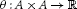and $a,b\in A$ with $\theta \left(a,b\right)\ne 0$. Suppose that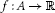is a differentiable function. If ${f}^{\mathrm{\prime }}$ is integrable on the θ-path ${P}_{bc},c=b+\theta \left(a,b\right)$ then, the following equality holds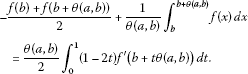(7)

Proof Suppose that $a,b\in A$. Since A is an invex set with respect to θ, for every $t\in \left[0,1\right]$ we have $b+t\theta \left(a,b\right)\in A$. Integrating by parts implies that(8)

which completes the proof. □

Theorem 2.1 Letbe an open invex subset with respect to. Suppose thatis a differentiable function. If $|{f}^{\mathrm{\prime }}|$ is preinvex on A then, for every $a,b\in A$ with $\theta \left(a,b\right)\ne 0$ the following inequality holds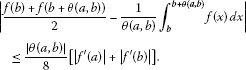(9)

Proof Suppose that $a,b\in A$. Since A is an invex set with respect to θ, for every $t\in \left[0,1\right]$ we have $b+t\theta \left(a,b\right)\in A$. By preinvexity of $|{f}^{\mathrm{\prime }}|$ and Lemma 2.1 we get(10)

where,

${\int }_{0}^{1}|1-2t|\left(1-t\right)\phantom{\rule{0.2em}{0ex}}dt={\int }_{0}^{1}|1-2t|t\phantom{\rule{0.2em}{0ex}}dt=\frac{1}{4}.$

□

Now, we give an example of an invex set with respect to an θ which is satisfies the conditions of Theorem 2.1.

Example 2.1 Suppose that $K:=\left(-3,-1\right)\cup \left(1,4\right)$ and the functionis defined by

$\theta \left(x,y\right)=\left\{\begin{array}{cc}x-y,\hfill & x>0,y>0,\hfill \\ x-y,\hfill & x<0,y<0,\hfill \\ 3-y,\hfill & x<0,y>0,\hfill \\ -2-y,\hfill & x>0,y<0.\hfill \end{array}$

Clearly K is an open invex set with respect to θ. Suppose that $a\in \left(-3,-1\right)$ and $b\in \left(1,4\right)$, $b\ne 3$ hence, $\theta \left(a,b\right)=3-b\ne 0$. Now,

${P}_{bc}=\left[b,3\right],\phantom{\rule{1em}{0ex}}b<3,$

and

${P}_{bc}=\left[3,b\right],\phantom{\rule{1em}{0ex}}b>3,$

where $c=b+\theta \left(a,b\right)$.

Another similar result is embodied in the following theorem.

Theorem 2.2 Letbe an open invex subset with respect to. Suppose thatis a differentiable function. Assume that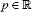with $p>1$. If $|{f}^{\mathrm{\prime }}{|}^{p/p-1}$ is preinvex on A then, for every $a,b\in A$ with $\theta \left(a,b\right)\ne 0$ the following inequality holds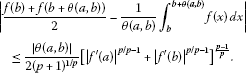(11)

Proof Suppose that $a,b\in A$. By assumption, Hölder’s inequality and the proof of Theorem 4.1 we have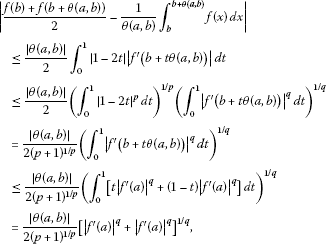(12)

where $q:=p/\left(p-1\right)$. □

Note that if $A=\left[a,b\right]$ and $\theta \left(x,y\right)=x-y$ for every $x,y\in A$ then, we can deduce Theorems 1.1 and 1.2, from Theorems 2.1 and 2.2, respectively.

## 3 An extension to several variables functions

The aim of this section is to extend the Proposition 1 in  and Theorem 2.2 to functions of several variables defined on invex subsets of.

The mappingis said to be satisfies the condition C if for every $x,y\in S$ and $t\in \left[0,1\right]$,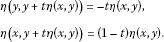Note that, in Example 2.1, θ satisfies the condition C.

For every $x,y\in S$ and every ${t}_{1},{t}_{2}\in \left[0,1\right]$ from condition C we have

$\eta \left(y+{t}_{2}\eta \left(x,y\right),y+{t}_{1}\eta \left(x,y\right)\right)=\left({t}_{2}-{t}_{1}\right)\eta \left(x,y\right),$
(13)

see  for details.

Proposition 3.1 Letbe an invex set with respect toand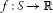is a function. Suppose that η satisfies condition C on S. Then, for every $x,y\in S$ the function f is preinvex with respect to η on η-path ${P}_{xv}$ if and only if the function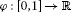defined by

$\phi \left(t\right):=f\left(x+t\eta \left(y,x\right)\right),$

is convex on $\left[0,1\right]$.

Proof Suppose that φ is convex on $\left[0,1\right]$ and ${z}_{1}:=x+{t}_{1}\eta \left(y,x\right)\in {P}_{xv}$, ${z}_{2}:=x+{t}_{2}\eta \left(y,x\right)\in {P}_{xv}$. Fix $\lambda \in \left[0,1\right]$. Since η satisfies condition C, by (13) we have

$\begin{array}{rcl}f\left({z}_{1}+\lambda \eta \left({z}_{2},{z}_{1}\right)\right)& =& f\left(x+\left(\left(1-\lambda \right){t}_{1}+\lambda {t}_{2}\right)\eta \left(y,x\right)\right)\\ =& \phi \left(\left(1-\lambda \right){t}_{1}+\lambda {t}_{2}\right)\\ \le & \left(1-\lambda \right)\phi \left({t}_{1}\right)+\lambda \phi \left({t}_{2}\right)\\ =& \left(1-\lambda \right)f\left({z}_{1}\right)+\lambda f\left({z}_{2}\right).\end{array}$
(14)

Hence, f is preinvex with respect to η on η-path ${P}_{xv}$.

Conversely, let $x,y\in S$ and the function f be preinvex with respect to η on η-path ${P}_{xv}$. Suppose that ${t}_{1},{t}_{2}\in \left[0,1\right]$. Then, for every $\lambda \in \left[0,1\right]$ we have

$\begin{array}{rcl}\phi \left(\left(1-\lambda \right){t}_{1}+\lambda {t}_{2}\right)& =& f\left(x+\left(\left(1-\lambda \right){t}_{1}+\lambda {t}_{2}\right)\eta \left(y,x\right)\right)\\ =& f\left(x+{t}_{1}\eta \left(y,x\right)\right)+\lambda \eta \left(x+{t}_{2}\eta \left(y,x\right),x+{t}_{1}\eta \left(y,x\right)\right)\\ \le & \lambda f\left(x+{t}_{2}\eta \left(y,x\right)\right)+\left(1-\lambda \right)f\left(x+{t}_{1}\eta \left(y,x\right)\right)\\ =& \lambda \phi \left({t}_{2}\right)+\left(1-\lambda \right)\phi \left({t}_{1}\right).\end{array}$
(15)

Therefore, φ is quasi-convex on $\left[0,1\right]$. □

The following theorem is a generalization of Proposition 1 in .

Theorem 3.1 Letbe an open invex set with respect to. Assume that η satisfies condition C. Suppose that for every $x,y\in S$ the functionis preinvex with respect to η on η-path ${P}_{xv}$. Then, for every $a,b\in \left(0,1\right)$ with $a the following inequality holds,(16)

Proof Let $x,y\in S$ and $a,b\in \left(0,1\right)$ with $a. Since f is preinvex with respect to η on η-path ${P}_{xv}$ by Proposition 3.1 the function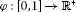defined by

$\phi \left(t\right):=f\left(x+t\eta \left(y,x\right)\right),$

is convex on $\left[0,1\right]$. Now, we define the function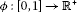as follows

$\varphi \left(t\right):={\int }_{0}^{t}\phi \left(s\right)\phantom{\rule{0.2em}{0ex}}ds={\int }_{0}^{t}f\left(x+s\eta \left(y,x\right)\right)\phantom{\rule{0.2em}{0ex}}ds.$

Obviously for every $t\in \left(0,1\right)$ we have

${\varphi }^{\mathrm{\prime }}\left(t\right)=\phi \left(t\right)=f\left(x+t\eta \left(y,x\right)\right)\ge 0,$

hence, $|{\varphi }^{\mathrm{\prime }}\left(t\right)|={\varphi }^{\mathrm{\prime }}\left(t\right)$ . Applying Theorem 1.1 to the function ϕ implies that

$|\frac{\varphi \left(a\right)+\varphi \left(b\right)}{2}-\frac{1}{b-a}{\int }_{a}^{b}\varphi \left(s\right)\phantom{\rule{0.2em}{0ex}}ds|\le \frac{\left(b-a\right)\left({\varphi }^{\mathrm{\prime }}\left(a\right)+{\varphi }^{\mathrm{\prime }}\left(b\right)\right)}{8},$

and we deduce that (16) holds. □

## 4 Second-order differentiable functions

In this section, we introduce some generalizations of Hermite-Hadamard-type inequality for functions whose second derivatives absolute values are preinvex. We begin with the following lemma (see Lemma 1 in  and Lemma 4 in ).

Lemma 4.1 Letbe an open invex subset with respect toand $a,b\in A$ with $\theta \left(a,b\right)\ne 0$. Suppose thatis a differentiable function. If ${f}^{\mathrm{\prime }\mathrm{\prime }}$ is integrable on the θ-path ${P}_{bc}$, $c=b+\theta \left(a,b\right)$ then, the following equality holds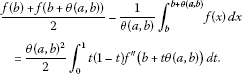(17)

Proof Suppose that $a,b\in A$. Since A is an invex set with respect to θ, for every $t\in \left[0,1\right]$ we have $b+t\theta \left(a,b\right)\in A$. Integrating by parts implies that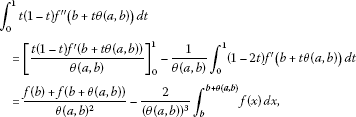(18)

which completes the proof. □

Theorem 4.1 Letbe an open invex subset with respect toand $\theta \left(a,b\right)\ne 0$ for all $a\ne b$. Suppose thatis a twice differentiable function on A. If $|{f}^{″}|$ is preinvex on A and ${f}^{″}$ is integrable on the θ-path ${P}_{bc}$, $c=b+\theta \left(a,b\right)$ then, the following inequality holds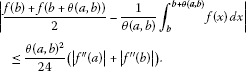(19)

Proof Suppose that $a,b\in A$. Since A is an invex set with respect to θ, for every $t\in \left[0,1\right]$ we have $b+t\theta \left(a,b\right)\in A$. By preinvexity of $|{f}^{″}|$ and Lemma 4.1 we get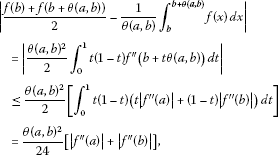(20)

which completes the proof. □

The corresponding version for powers of the absolute value of the second derivative is incorporated in the following theorem.

Theorem 4.2 Letbe an open invex subset with respect toand $\theta \left(a,b\right)\ne 0$ for all $a\ne b$. Suppose thatis a twice differentiable function on A and ${|{f}^{″}|}^{\frac{p}{p-1}}$ is preinvex on A, for $p>1$. If ${f}^{″}$ is integrable on the θ-path ${P}_{bc}$, $c=b+\theta \left(a,b\right)$ then, the following inequality holds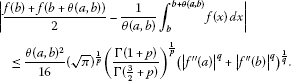(21)

Proof By preinvexity of $|{f}^{″}|$, Lemma 4.1 and using the well-known Hölder integral inequality, we get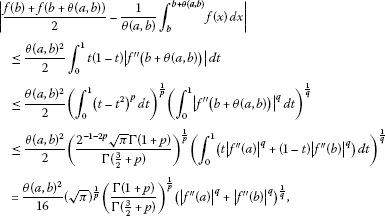which completes the proof. □

A more general inequality is given using Lemma 4.1, as follows:

Theorem 4.3 Letbe an open invex subset with respect toand $\theta \left(a,b\right)\ne 0$ for all $a\ne b$. Suppose thatis a twice differentiable function on A and ${|{f}^{″}|}^{q}$ is preinvex on A, for $q>1$. If ${f}^{″}$ is integrable on the θ-path ${P}_{bc}$, $c=b+\theta \left(a,b\right)$ then, the following inequality holds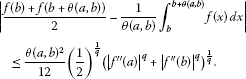(22)

Proof By preinvexity of $|{f}^{″}|$, Lemma 4.1 and using the well-known weighted power mean inequality, we get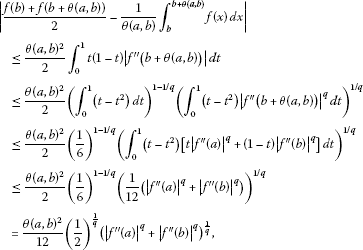which completes the proof. □

## References

1. Dragomir SS: Two mappings on connection to Hadamard’s inequality. J. Math. Anal. Appl. 1992, 167: 49–56. 10.1016/0022-247X(92)90233-4

2. Dragomir SS: On Hadamard’s inequalities for convex functions. Math. Balk. 1992, 6: 215–222.

3. Dragomir SS, Pecaric JE, Sandor J: A note on the Jensen-Hadamard inequality. Anal. Numér. Théor. Approx. 1990, 19: 29–34.

4. Dragomir SS, Pecaric JE, Persson LE: Some inequalities of Hadamard type. Soochow J. Math. 1995, 21: 335–341.

5. Dragomir SS, Agarwal RP: Two inequalities for differentiable mappings and applications to special means of real numbers and to trapezoidal formula. Appl. Math. Lett. 1998, 11: 91–95.

6. Ion DA: Some estimates on the Hermite-Hadamard inequality through quasiconvex functions. Ann. Univ. Craiova, Math. Comput. Sci. Ser. 2007, 34: 82–87.

7. Hanson MA: On sufficiency of the Kuhn-Tucker conditions. J. Math. Anal. Appl. 1981, 80: 545–550. 10.1016/0022-247X(81)90123-2

8. Weir T, Mond B: Preinvex functions in multiple objective optimization. J. Math. Anal. Appl. 1998, 136: 29–38.

9. Noor MA: Hermite-Hadamard integral inequalities for log-preinvex functions. J. Math. Anal. Approx. Theory 2007, 2: 126–131.

10. Noor MA: On Hadamard integral inequalities involving two log-preinvex functions. J. Inequal. Pure Appl. Math. 2007, 8: 1–6.

11. Antczak T: Mean value in invexity analysis. Nonlinear Anal. 2005, 60: 1471–1484.

12. Yang XM, Li D: On properties of preinvex functions. J. Math. Anal. Appl. 2001, 256: 229–241. 10.1006/jmaa.2000.7310

13. Mohan SR, Neogy SK: On invex sets and preinvex function. J. Math. Anal. Appl. 1995, 189: 901–908. 10.1006/jmaa.1995.1057

14. Alomari M, Drus M, Dragomir SS: New inequalities of Hermite-Hadamard type for functions whose second derivatives absolute values are quasi-convex. Tamkang J. Math. 2010, 41: 353–359.

15. Dragomir SS, Pearce CEM RGMIA Monographs. In Selected Topics on Hermite-Hadamard Inequalities and Applications. Victoria University, Melbourne; 2000. http://ajmaa.org/RGMIA/monographs.php

## Author information

Authors

### Corresponding author

Correspondence to Ali Barani.

### Competing interests

The authors declare that they have no competing interests.

### Authors’ contributions

All authors conceived of the study, and participated in the design and coordination. All authors read and approved the final manuscript.

## Rights and permissions

Reprints and Permissions

Barani, A., Ghazanfari, A.G. & Dragomir, S.S. Hermite-Hadamard inequality for functions whose derivatives absolute values are preinvex. J Inequal Appl 2012, 247 (2012). https://doi.org/10.1186/1029-242X-2012-247